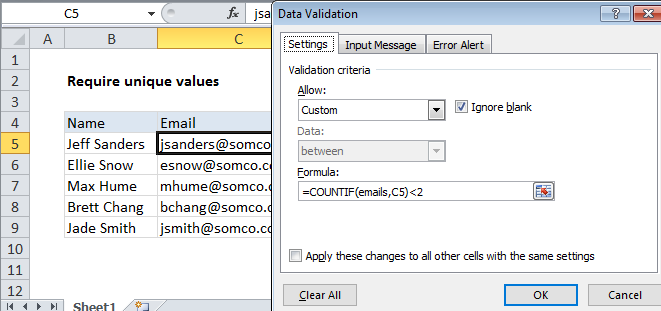## Excel Office

Excel How Tos, Tutorials, Tips & Tricks, Shortcuts

# Reject duplicate data entry in a cell range.

To allow only unique values in a given range, you can use data validation with a custom formula based on the COUNTIF function.

## Formula

`=COUNTIF(range,A1)<2`

##Note: Cell references in data validation formulas are relative to the upper left cell in the range selected when the validation rule is defined, in this case B5.

Also See:   Count missing values in Excel

## Explanation

In the example shown, the data validation applied to C5:C9 is:

`=COUNTIF(emails,C5)<2`

where emails is the named range C5:C9.

### How this formula works

Data validation rules are triggered when a user adds or changes a cell value. In this example, we are using a formula that checks that the input doesn’t already exist in the named range “emails”:

`COUNTIF(ids,B5)<2`

COUNTIF returns a count of the value in C5 inside the named range emails (C5:C9). If the count is less than 2, the expression returns TRUE and validation succeeds. If not, the expression returns FALSE and validation fails.

Also See:   Count cells less than in Excel